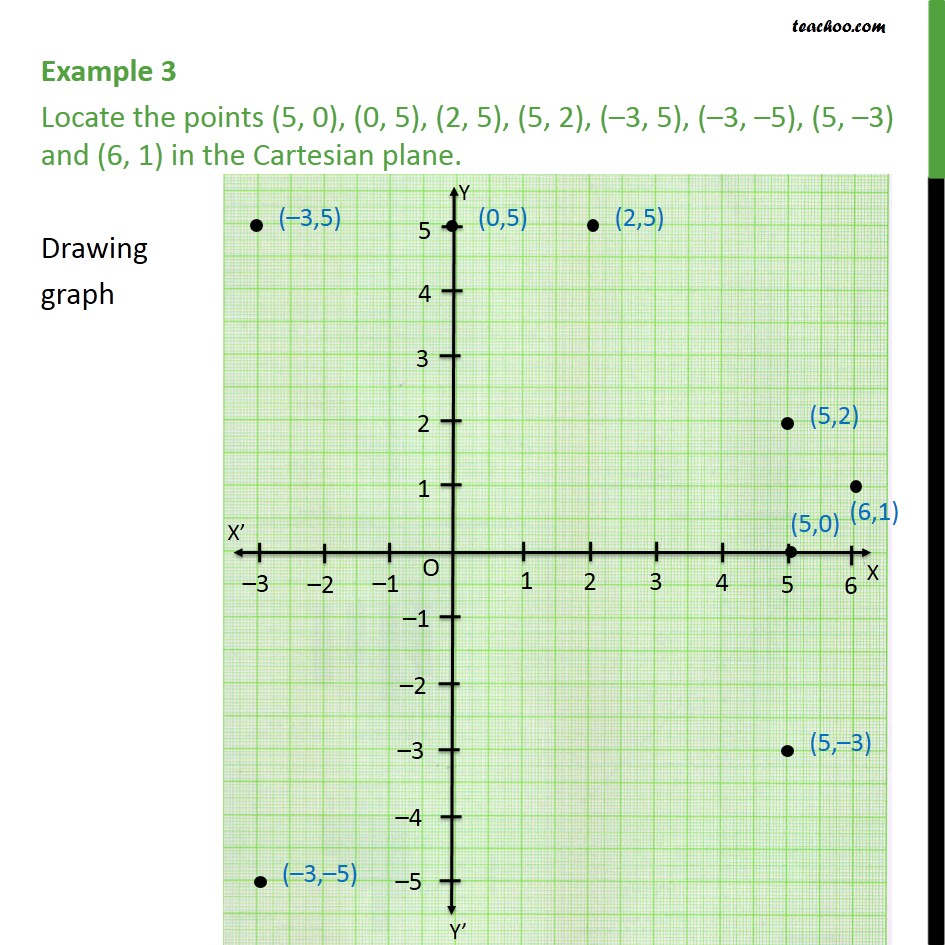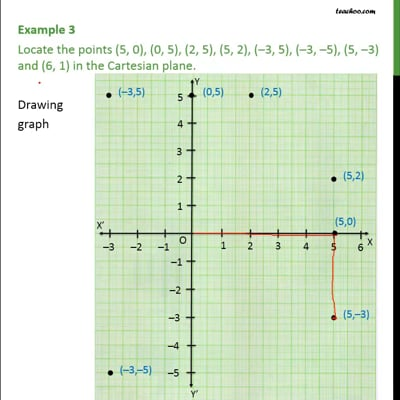Chapter 3 Class 9 Coordinate Geometry

Class 9
Important Questions for Exam - Class 9This video is only available for Teachoo black users

Introducing your new favourite teacher - Teachoo Black, at only ₹83 per month

### Transcript

Example 3 Locate the points (5, 0), (0, 5), (2, 5), (5, 2), (–3, 5), (–3, –5), (5, –3) and (6, 1) in the Cartesian plane. We follow these steps to mark points 1. Drawing x and y axis 2. Now, in all points, maximum value of x is 6, and minimum is -3. So, we draw x-axis from -3 to 6 3. Also, in all points, maximum value of y is 5, and minimum is -3. So, we draw y-axis from -3 to 5 4. Let's mark points one by one 5. For (5, 0), we go to 5 in x-axis. Now, since y is 0. We mark that point. 6. Similarly, we can plot all points